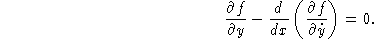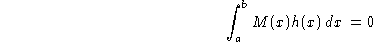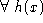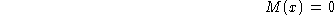Next: Calculus of Variations: Distance Between Up: c Previous: Calculus Fundamental Theorem (Second)

## Calculus of Variations

A branch of mathematics which is a sort of generalization of Calculus. Calculus of variations seeks to find the path, curve, surface, etc., for which a given Function has a Stationary Value (which, in physical problems, is usually a Minimum or Maximum). Mathematically, this involves finding Stationary Values of integrals of the formI has an extremum only if the Euler-Lagrange Differential Equation is satisfied, i.e., ifThe Calculus of Variations Fundamental Lemma states that, ifwith Continuous second Partial Derivatives, thenon (a,b).

See also Beltrami Identity, Bolza Problem, Brachistochrone Problem, Catenary, Envelope Theorem, Euler-Lagrange Differential Equation, Isoperimetric Problem, Isovolume Problem, Lindelof's Theorem, Partition Calculus, Plateau's Problem, Roulette, Skew Quadrilateral, Sphere with Tunnel, Unduloid, Weierstraß-Erdman Corner Condition

ReferencesCalculus of Variations